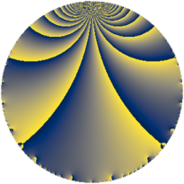Properties

 Label 3640.2.a.nLevel $3640$ Weight $2$ Character orbit 3640.a Self dual yes Analytic conductor $29.066$ Analytic rank $1$ Dimension $2$ CM no Inner twists $1$

Related objects

Newspace parameters

 Level: $$N$$ $$=$$ $$3640 = 2^{3} \cdot 5 \cdot 7 \cdot 13$$ Weight: $$k$$ $$=$$ $$2$$ Character orbit: $$[\chi]$$ $$=$$ 3640.a (trivial)

Newform invariants

 Self dual: yes Analytic conductor: $$29.0655463357$$ Analytic rank: $$1$$ Dimension: $$2$$ Coefficient field: $$\Q(\sqrt{5})$$ Defining polynomial: $$x^{2} - x - 1$$ Coefficient ring: $$\Z[a_1, a_2, a_3]$$ Coefficient ring index: $$1$$ Twist minimal: yes Fricke sign: $$1$$ Sato-Tate group: $\mathrm{SU}(2)$

$q$-expansion

Coefficients of the $$q$$-expansion are expressed in terms of $$\beta = \frac{1}{2}(1 + \sqrt{5})$$. We also show the integral $$q$$-expansion of the trace form.

 $$f(q)$$ $$=$$ $$q + \beta q^{3} + q^{5} + q^{7} + ( -2 + \beta ) q^{9} +O(q^{10})$$ $$q + \beta q^{3} + q^{5} + q^{7} + ( -2 + \beta ) q^{9} -4 \beta q^{11} + q^{13} + \beta q^{15} + ( -3 - \beta ) q^{17} + ( -1 - \beta ) q^{19} + \beta q^{21} + ( -6 + 4 \beta ) q^{23} + q^{25} + ( 1 - 4 \beta ) q^{27} + ( -4 + \beta ) q^{29} + 5 \beta q^{31} + ( -4 - 4 \beta ) q^{33} + q^{35} + ( 4 - 5 \beta ) q^{37} + \beta q^{39} + ( -7 - \beta ) q^{41} + ( -4 + 2 \beta ) q^{43} + ( -2 + \beta ) q^{45} + ( -2 + 4 \beta ) q^{47} + q^{49} + ( -1 - 4 \beta ) q^{51} + ( 2 - 4 \beta ) q^{53} -4 \beta q^{55} + ( -1 - 2 \beta ) q^{57} + ( 8 - 9 \beta ) q^{59} + ( -10 + 4 \beta ) q^{61} + ( -2 + \beta ) q^{63} + q^{65} + ( -1 - \beta ) q^{67} + ( 4 - 2 \beta ) q^{69} + ( -12 + 6 \beta ) q^{71} + ( -4 + 4 \beta ) q^{73} + \beta q^{75} -4 \beta q^{77} + ( 11 - 9 \beta ) q^{79} + ( 2 - 6 \beta ) q^{81} -6 q^{83} + ( -3 - \beta ) q^{85} + ( 1 - 3 \beta ) q^{87} + ( -4 + 7 \beta ) q^{89} + q^{91} + ( 5 + 5 \beta ) q^{93} + ( -1 - \beta ) q^{95} + ( -6 - 6 \beta ) q^{97} + ( -4 + 4 \beta ) q^{99} +O(q^{100})$$ $$\operatorname{Tr}(f)(q)$$ $$=$$ $$2 q + q^{3} + 2 q^{5} + 2 q^{7} - 3 q^{9} + O(q^{10})$$ $$2 q + q^{3} + 2 q^{5} + 2 q^{7} - 3 q^{9} - 4 q^{11} + 2 q^{13} + q^{15} - 7 q^{17} - 3 q^{19} + q^{21} - 8 q^{23} + 2 q^{25} - 2 q^{27} - 7 q^{29} + 5 q^{31} - 12 q^{33} + 2 q^{35} + 3 q^{37} + q^{39} - 15 q^{41} - 6 q^{43} - 3 q^{45} + 2 q^{49} - 6 q^{51} - 4 q^{55} - 4 q^{57} + 7 q^{59} - 16 q^{61} - 3 q^{63} + 2 q^{65} - 3 q^{67} + 6 q^{69} - 18 q^{71} - 4 q^{73} + q^{75} - 4 q^{77} + 13 q^{79} - 2 q^{81} - 12 q^{83} - 7 q^{85} - q^{87} - q^{89} + 2 q^{91} + 15 q^{93} - 3 q^{95} - 18 q^{97} - 4 q^{99} + O(q^{100})$$

Embeddings

For each embedding $$\iota_m$$ of the coefficient field, the values $$\iota_m(a_n)$$ are shown below.

For more information on an embedded modular form you can click on its label.

Label $$\iota_m(\nu)$$ $$a_{2}$$ $$a_{3}$$ $$a_{4}$$ $$a_{5}$$ $$a_{6}$$ $$a_{7}$$ $$a_{8}$$ $$a_{9}$$ $$a_{10}$$
1.1
 −0.618034 1.61803
0 −0.618034 0 1.00000 0 1.00000 0 −2.61803 0
1.2 0 1.61803 0 1.00000 0 1.00000 0 −0.381966 0
 $$n$$: e.g. 2-40 or 990-1000 Significant digits: Format: Complex embeddings Normalized embeddings Satake parameters Satake angles

Atkin-Lehner signs

$$p$$ Sign
$$2$$ $$-1$$
$$5$$ $$-1$$
$$7$$ $$-1$$
$$13$$ $$-1$$

Inner twists

This newform does not admit any (nontrivial) inner twists.

Twists

By twisting character orbit
Char Parity Ord Mult Type Twist Min Dim
1.a even 1 1 trivial 3640.2.a.n 2
4.b odd 2 1 7280.2.a.z 2

By twisted newform orbit
Twist Min Dim Char Parity Ord Mult Type
3640.2.a.n 2 1.a even 1 1 trivial
7280.2.a.z 2 4.b odd 2 1

Hecke kernels

This newform subspace can be constructed as the intersection of the kernels of the following linear operators acting on $$S_{2}^{\mathrm{new}}(\Gamma_0(3640))$$:

 $$T_{3}^{2} - T_{3} - 1$$ $$T_{11}^{2} + 4 T_{11} - 16$$

Hecke characteristic polynomials

$p$ $F_p(T)$
$2$ $$T^{2}$$
$3$ $$-1 - T + T^{2}$$
$5$ $$( -1 + T )^{2}$$
$7$ $$( -1 + T )^{2}$$
$11$ $$-16 + 4 T + T^{2}$$
$13$ $$( -1 + T )^{2}$$
$17$ $$11 + 7 T + T^{2}$$
$19$ $$1 + 3 T + T^{2}$$
$23$ $$-4 + 8 T + T^{2}$$
$29$ $$11 + 7 T + T^{2}$$
$31$ $$-25 - 5 T + T^{2}$$
$37$ $$-29 - 3 T + T^{2}$$
$41$ $$55 + 15 T + T^{2}$$
$43$ $$4 + 6 T + T^{2}$$
$47$ $$-20 + T^{2}$$
$53$ $$-20 + T^{2}$$
$59$ $$-89 - 7 T + T^{2}$$
$61$ $$44 + 16 T + T^{2}$$
$67$ $$1 + 3 T + T^{2}$$
$71$ $$36 + 18 T + T^{2}$$
$73$ $$-16 + 4 T + T^{2}$$
$79$ $$-59 - 13 T + T^{2}$$
$83$ $$( 6 + T )^{2}$$
$89$ $$-61 + T + T^{2}$$
$97$ $$36 + 18 T + T^{2}$$